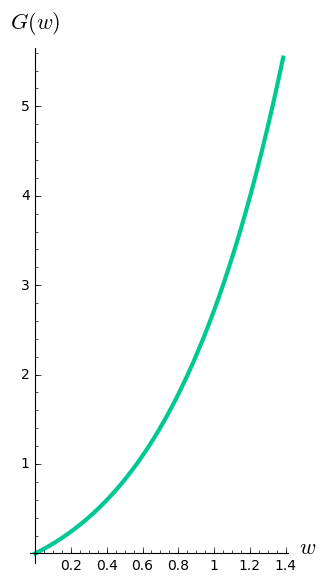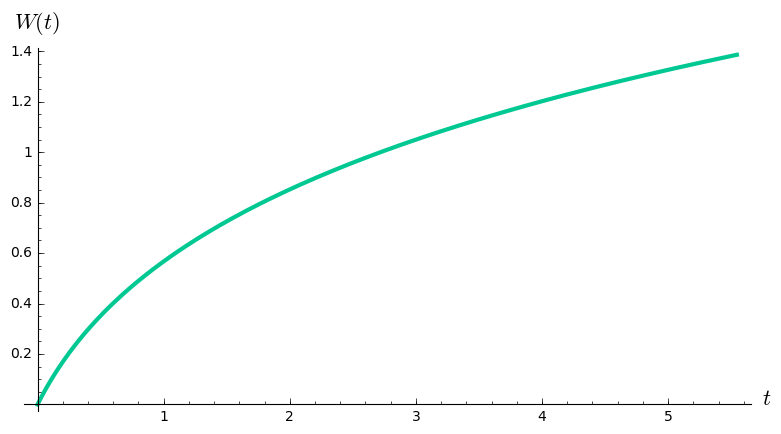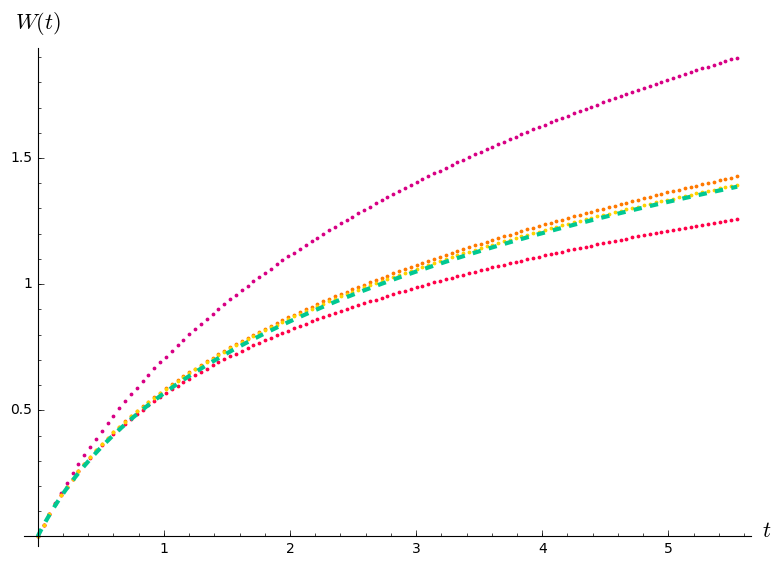# Approximating solutions¶

In :
import numpy
w = var('w')
palette = [(215/255, 0/255, 132/255), (255/255, 1/255, 73/255), (255/255, 121/255, 1/255), (255/255, 210/255, 0/255)]
cool_palette = [(0/255, 150/255, 173/255), (0/255, 200/255, 146/255)]


## Computing a special function with Picard iteration¶

The function $G(w) = w e^w$ is invertible on the domain $[0, \infty)$.

In :
g_graph = plot(w*exp(w), (w, 0, log(4)), thickness = 3, color = cool_palette)
g_labels = ['$w$', '$G(w)$']
show(g_graph, axes_labels = g_labels, aspect_ratio = 1/2)Its inverse $W(t)$ is called the "Lambert $W$ function." We can draw a graph of $W$ by graphing $G$ and then reflecting to switch the input and the output. If we have a way to calculate $e^w$, we can use this idea to calculate $W$. But could we calculate $W$ directly?

In :
w_graph = parametric_plot((w*exp(w), w), (w, 0, log(4)), thickness = 3, color = cool_palette)
w_labels = ['$t$', '$W(t)$']
show(w_graph, aspect_ratio = 2, axes_labels = w_labels)Even if we don't know how to calculate $e^w$, there's one value of $G$ we can calculate: we know $G(0) = 0$. Flipping inputs and outputs, we learn that $W(0) = 0$ too.

We know from the definition of an inverse that $G(W(t)) = t$. In other words $W(t) e^{W(t)} = t$. Differentiating both sides with respect to $t$, and using the definition of $W$, you can deduce that $$W'(t) = \frac{1}{t + e^{W(t)}}.$$ Now we have a differential equation for $W$, and the initial value information $W(0) = 0$, so we can approximate $W$ using Picard iteration.

Let's try it, approximating the integral with a 120-step Riemann sum. By the fourth iteration after our constant initial guess, the graph of the approximation is so close to the flipped graph of $G$ that the difference is hard to see.

In :
t_grid = numpy.linspace(0, N(log(4)*4), 121)
w_appx = [numpy.empty(len(t_grid))]
w_appx.fill(0)
for _ in range(4):
next_appx = numpy.cumsum([t_grid / (t_val + exp(w_val)) for (t_val, w_val) in zip(t_grid, w_appx[-1][0:-1])])
w_appx.append(numpy.insert(next_appx, 0, 0))
dashed_w_graph = parametric_plot((w*exp(w), w), (w, 0, log(4)), thickness = 3, color = cool_palette, linestyle = 'dashed')
picard_iterates = sum([scatter_plot(zip(t_grid, w_appx[k+1]), edgecolor = palette[k], facecolor = palette[k], markersize = 3, zorder = 0) for k in range(4)])
show(dashed_w_graph + picard_iterates, aspect_ratio = 2, axes_labels = w_labels)